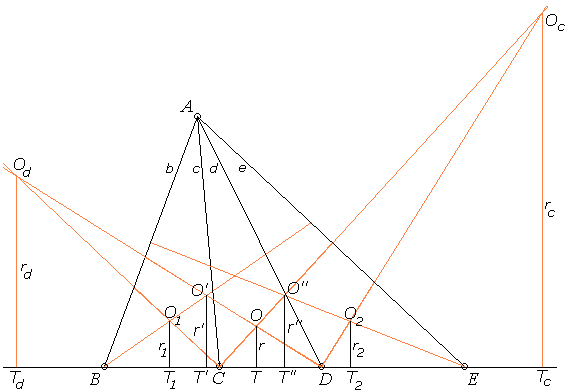### This applet requires Sun's Java VM 2 which your browser may perceive as a popup. Which it is not. If you want to see the applet work, visit Sun's website at https://www.java.com/en/download/index.jsp, download and install Java VM and enjoy the applet.

 What if applet does not run?

Explanation### Five Incircles Theorem

The applet suggests the following theorem:

 (A) In ΔABE two cevians AC and AD are drawn from the apex A to the base BE. Let the points be located as in the diagram below. Then the incircles of triangles ABC and ADE are equal iff the incircles of triangles ABD and ACE are equal.The theorem and the proof are due to J. B. Tabov, (Bulgaria) who responded to the challenge issued by H. Demir (Turkey) to provide a geometric proof (the original proof was trigonometric) to the following statement

 (B) Assume under the conditions of (A), triangles ABC, ACD, and ADE have equal incircles. Then the incircles of triangles ABD and ACE are equal.

(B) is clearly more restrictive and its assertion is less satisfying.

### Proof of (A)

Since triangles ODT and OdDTd are similar, r/rd = DT/DTd. And, Since triangles O1CT1 and OdCTd are similar, r1/rd = CT1/CTd. Combining the two proportions we get

 (1) r/r1 = DT/DTd · CTd/CT1.

Now, in addition to the notions used in the diagram, denote for convenience CD = a, and the semiperimeters of triangles ABC, ACD, and ADE, respectively, s1, s, s2. Then, DT = s - c, DTd = s (this is because Od is the center of an excircle of ΔACD), CTd = DTd - CD = s - a, and, finally, CT1 = s1 - b. (1) then becomes

r/r1 = (s - c)/s · (s - a)/(s1 - b).

Similarly,

r/r1 = (s - d)/s · (s - a)/(s2 - e).

Hence r1 = r2 iff

 (2) (s - d)(s1 - b) = (s - c)(s2 - e).

We can do something similar for triangles ABD and ACE. Indeed, since triangles ODT and O'DT' are similar, r'/r = DT'/DT. Since triangles OCT and O"CT" are similar, r"/r = CT"/CT. Therefore, r' = r" iff

 (3) (s - d)(s' - b) = (s - c)(s" - e),

where s' and s" are the semiperimeters of triangles ABD and ACE, respectively. But clearly s' = s + s1 - c and s" = s + s2 - d, so that

 (s - d)(s' - b) - (s - c)(s" - e) = (s - d)(s + s1 - c - b) - (s - c)(s + s2 - d - e) = (s - d)(s - c) + (s - d)(s1 - b) - (s - c)(s - d) - (s - c)(s2 - e) = (s - d)(s1 - b) - (s - c)(s2 - e)

Consequently, (2) and (3) are equivalent, which proves the theorem.

The theorem has bearing on the Equal Incircles Theorem. A more general statement admits even a simpler proof.

### References

1. H. Demir, Incircles within, Math Magazine, v 59 (1986), 77-83
2. J. B. Tabov, A Note on the Five-Circle Theorem, Math Magazine, v 63 (1990), 92-94